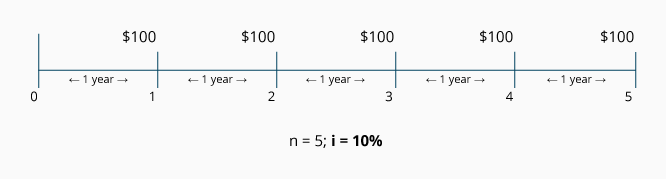## Introduction to the Present Value of an Ordinary Annuity

Suppose a business owes you \$3,000 and offers you two repayment choices: (1) it will give you three payments of \$1,000 each at the end of years 2023, 2024, and 2025, or (2) it will give you the total \$3,000 at the beginning of the year 2023. Which repayment option should you choose? To maximize your dollars, you should choose the one that gives you the largest present value—in this case, option #2.

If you receive and invest \$100 today, it will grow over time to be worth more than \$100. This fact of financial life is a result of the time value of money, a concept which says it's more valuable to receive \$100 now rather than a year from now. It also means that receiving \$100 one year from now is less valuable than receiving that same \$100 today. In other words, the \$100 received one year from now has a present value that is smaller than \$100.

### Ordinary Annuity

An annuity is a series of identical payments occurring at equal time intervals. When the payments appear at the end of each time period, the annuity is said to be an ordinary annuity or an annuity in arrears. The following timeline depicts an ordinary annuity comprised of five payments of \$100 each:The equal periods of time (represented by n) between the identical payments of \$100 could be a year, a 6-month period, a quarter of a year, a month, etc. In the above example, n = 5 periods of one year each. The interest rate (represented by i) is used to discount the \$100 payments to time period 0. The interest rate might be the company's required rate, its target rate, its cost of capital, etc. The \$100 amounts are often represented by either the letters "PMT" (for "payment" or receipt) or as "rent" (since the amount is the same each period).

We are going to focus here on ordinary annuities—annuities with equal payments occurring at the end of each period (as shown in the above timeline). Annuities due or annuities in advance—annuities with the equal payment amounts occurring at the beginning of each period—will be another topic.)

### Accounting Applications

Accountants use present value calculations of an ordinary annuity in a number of applications. For example:

1. Your company provides a service in December 2022 and agrees to be paid in three installments of \$100 each. The payments will occur at the end of each of the years 2023, 2024, and 2025. The time value of money tells us that a portion of the three \$100 payments represents interest your company will earn because it has agreed to wait for its money. It may be that only \$250 of the total \$300 in payments can be considered service revenue earned in 2022; the remaining \$50 is interest revenue earned over the three years 2023, 2024, and 2025. How do you determine what amount is actual service revenue and what amount is implicit interest revenue? By using a present value calculation, you can remove the implicit interest so that the amount of service revenue can be determined.

2. Your company is interested in buying the parcel of land adjacent to its present site. The owners will either sell the land to you for \$380,000 today, or they will sell it to you for five payments of \$100,000 each over the next five years. (The payments will be one year apart with the first payment due one year from now.) Which arrangement makes the most financial sense to your company? By using a present value calculation, you can determine the interest rate implicit in the five-payment arrangement.

In addition to these two examples, we will see that present value calculations can also tell you such things as how much money to invest now in return for specific cash amounts to be received in the future, and how to estimate the rate of return on your investments. Our focus throughout this topic will be on ordinary annuities—streams of equal cash amounts that are received or paid at the end of future periods. We'll discuss calculations that determine present value, interest rate, and/or the length of time needed for identical payments to occur.

#### Here's a Tip

You may find it helpful to read our explanation Present Value of a Single Amount.

## Components of a Present Value Calculation

In present value calculations, future cash amounts are discounted back to the present time. ("Discounting" means removing the interest that is imbedded in the future cash amounts.) As a result, present value calculations are often referred to as a discounted cash flow technique. It's important for you to understand that present value calculations involve cash amounts—not accrual accounting amounts.

Present value calculations involve the compounding of interest. This means that any interest earned is reinvested and will earn interest at the same rate as the principal. In other words, you earn "interest on interest." The compounding of interest can be very significant when the interest rate and/or the number of years are sizable.

The present value of an annuity (i.e., series of equal payments, receipts, rents) involves five components:

1. Present value
2. Amount of each identical cash payment
3. Time between the identical cash payments
4. Number of periods that the payments will occur; length of the annuity
5. Interest rate or target rate used for discounting the series of payments*

* This rate (represented by i) could be a loan interest rate, but it could also be a company's minimum rate of return to be earned on each investment.

If you know any four of these five components, you will be able to calculate the unknown component.